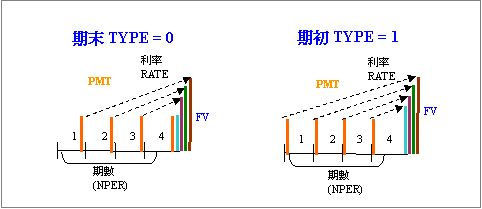﻿

基本概念

單筆及定期投資Excel的財務函數是將這兩種混合在一起，所以參數看起來較為複雜容易用錯，但只要了解以後用起來是既簡潔又方便。

定期投資的期初及期末現金流入及流出

Excel財務函數金額部分有正負號之分，正值代表金額流入，負數代表金額流出。例如你要計算整存整付定存的期末領回本利和。期初金額(PV)是由你交給銀行(現金流出所以是負值)，期滿後銀行會給付本利和的金額(現金流入所以是正值)。相反的如果是以銀行的角度來看，期初金額(PV)因為存款流入所以是正值，期滿算出的未來值(FV)應該是負值，代表要給付存款人的金額。

參數介紹

 函數 功能 參數 FV 到期後未來值(終值) =FV(rate, nper, pmt, [pv], [type]) PV 單筆的現值 =PV(rate, nper, pmt, [fv], [type]) RATE 每期的利率或報酬率 =RATE(nper, pmt, pv, [fv], [type], [guess]) PMT 每期投資金額 =PMT(rate, nper, pv, [fv], [type]) NPER 期數 =NPER(rate, pmt, pv, [fv], [type])

未來值Future Value(FV)

整存整付定存

RATE = 5% / 12 (每月為一期，月利率 = 年利率 / 12)
NPER = 6(半年分6期)
PMT = 0 (只有單筆所以設定0)
PV = -1,000,000(存入銀行一百萬，現金流出所以為負值)

=FV(5%/12, 6, 0, -1000000)
= \$1,025,262

零存整付定存

RATE = 4.5% / 12 (每月為一期，月利率 = 年利率 / 12)
NPER = 12(分12期)
PMT = -10,000(每月定期一萬元，因為現金流出所以負值)
PV = 0 (其初沒有單筆投入，所以等於零)

=FV(4.5%/12, 12, -10000, 0, 1)
= \$122,966。

預估投資收益

RATE = 6%/12(每月為一期，月利率 = 年利率 / 12)
NPER = 12*(60-37)(投資期數 276期)
PMT = -30,000(每月投資3萬元)
PV = -2,000,000(期初投200萬元)

=FV(6%/12, 12*(60-37), -30000, -2000000, 1)
= \$25,778,895

=FV(8%/12, 12*(60-37), -30000, -2000000, 1)
= \$36,336,094。

現值Present Value (PV)

退休金的現值

RATE = 2%(一期為一年，每年以2%成長)
NPER = 5(一年一期，所以期數等於5)
PAYMENT = 0(只有單筆，所以為0)
FV = 2,000,000(期末拿到兩百萬退休金)

=PV(2%, 5, 0, 2000000)
= -1,811,461

債券的價值

RATE:= 5% / 2(每半年為一期，每期利率為年利率除以2)
NPER = 7 (三年半，每半年一期總共七期)
PAYMENT = 30,000(每半年定期領息三萬元)
FV = 1,000,000(到期領回一百萬)

=PV(5%/2, 7, 30000, 1000000)
= -1,031,747

利率(RATE)

汽車貸款利率

NPER = 36 (每月一期分36期支付)
PMT= -\$19,360(每期支付19,360)
PV = 600,000(貸款60萬)

=RATE(36, -19360, 600000)
= 0.83324094142316%

投資年化報酬率 I

NPER = 5(每年為一期，分5期計算)
PMT = 0(每期沒有投資，所以為0)
PV = -\$100,000(期初投資10萬元，現金流出)
FV = \$220,000(期末淨值22萬元，現金流入)

=RATE(5, 0, -100000, 220000)
= 17.08%

投資年化報酬率 II

NPER = 5*12(每月為一期，分60期計算)
PMT = -10,000(每月投資10,000，現金流出)
PV = 0(期初沒有單筆投資)
FV = \$800,000(期末淨值80萬元)

=RATE(5*12, -10000, 0, 800000,1)*12
= 10.89%

每期投資金額PAYMENT (PMT)

本息定額償還貸款

RATE = 3.5%/12(每月為一期，月利率 = 年利率 / 12)
NPER = 20*12(分20*12 = 240期償還)
PV = \$3,500,000(貸款金額350萬)

=PMT(3.5%/12, 20*12, 3500000)
= -\$20,299

退休規劃

RATE = 6%/12(每月為一期，月利率 = 年利率 / 12)
NPER = 15*12(分15*12 = 180期投資)
PV = -\$2,000,000(期初投資200萬)
FV = \$10,000,000(期末金額1000萬)

=PMT(6%/12, 15*12, -2000000, 10000000)
= -17,509

期數(NPER)

退休規劃

RATE = 6%/12(每月為一期，月利率 = 年利率 / 12)
PMT = -50,000(每月投資5萬元)
PV = \$-2,000,000(已有200萬)
FV = \$10,000,000(期末金額1000萬)

=NPER(6%/12, -50000, -2000000, 10000000)
= 103期

延伸閱讀

Excel函數-PV現值

﻿

■ 本網站內容儘可能精確完整，但不保證無誤。若做為投資依據，風險請自行斟酌 ，本網站不負賠償之責任。
■ 網站所有資料均為版權所有，非經書面允許請勿轉載或使用。